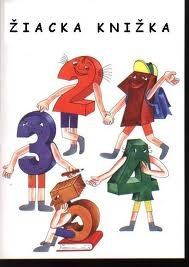# Mathematics 6522

There are more than 20 but less than 40 students in the class. A third of the pupils wrote the mathematics test with a one, a sixth with a two, and a ninth with a three. Nobody got a four. How many students in the class wrote the test on a five?

x =  36

### Step-by-step explanation:Did you find an error or inaccuracy? Feel free to write us. Thank you!

Tips for related online calculators
Do you want to calculate the least common multiple of two or more numbers?
Do you want to calculate the greatest common divisor of two or more numbers?
Need help calculating sum, simplifying, or multiplying fractions? Try our fraction calculator.